Applied Cryptography, Second Edition: Protocols, Algorthms, and Source Code in C (cloth)
(Publisher: John Wiley & Sons, Inc.)
Author(s): Bruce Schneier
ISBN: 0471128457
Publication Date: 01/01/96

Generalized Geffe Generator

Instead of choosing between two LFSRs, this scheme chooses between k LFSRs, as long as k is a power of 2. There are k + 1 LFSRs total (see Figure 16.7). LFSR-1 must be clocked log2k times faster than the other k LFSRs.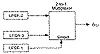Figure 16.6  Geffe generator.

Even though this scheme is more complex than the Geffe generator, the same kind of correlation attack is possible. I don’t recommend this generator.

Jennings Generator

This scheme uses a multiplexer to combine two LFSRs [778,779,780]. The multiplexer, controlled by LFSR-1, selects 1 bit of LFSR-2 for each output bit. There is also a function that maps the output of LFSR-2 to the input of the multiplexer (see Figure 16.8).

The key is the initial state of the two LFSRs and the mapping function. Although this generator has great statistical properties, it fell to Ross Anderson’s meet-in-the-middle consistency attack  and the linear consistency attack [1638,442]. Don’t use this generator.

Beth-Piper Stop-and-Go Generator

This generator, shown in Figure 16.9, uses the output of one LFSR to control the clock of another LFSR . The clock input of LFSR-2 is controlled by the output of LFSR-1, so that LFSR-2 can change its state at time t only if the output of LFSR-1 was 1 at time t - 1.

No one has been able to prove results about this generator’s linear complexity in the general case. However, it falls to a correlation attack .

Alternating Stop-and-Go Generator

This generator uses three LFSRs of different length. LFSR-2 is clocked when the output of LFSR-1 is 1; LFSR-3 is clocked when the output of LFSR-1 is 0. The output of the generator is the XOR of LFSR-2 and LFSR-3 (see Figure 16.10) .

This generator has a long period and large linear complexity. The authors found a correlation attack against LFSR-1, but it does not substantially weaken the generator. There have been other attempts at keystream generators along these lines [1534,1574,1477].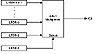Figure 16.7  Generalized Geffe generator.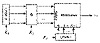Figure 16.8  Jennings generator.

Bilateral Stop-and-Go Generator

This generator uses two LFSRs, both of length n (see Figure 16.11) . The output of the generator is the XOR of the outputs of each LFSR. If the output of LFSR-2 at time t – 1 is 0 and the output at time t – 2 is 1, then LFSR-2 does not clock at time t. Conversely, if the output of LFSR-1 at time t – 1 is 0 and the output at t – 2 is 1, and if LFSR-1 clocked at time t, then LFSR-2 does not clock at time t.

The linear complexity of this system is roughly equal to the period. According to , “no evident key redundancy has been observed in this system.”

Threshold Generator

This generator tries to get around the security problems of the previous generators by using a variable number of LFSRs . The theory is that if you use a lot of LFSRs, it’s harder to break the cipher.

This generator is illustrated in Figure 16.12. Take the output of a large number of LFSRs (use an odd number of them). Make sure the lengths of all the LFSRs are relatively prime and all the feedback polynomials are primitive: maximize the period. If more than half the output bits are 1, then the output of the generator is 1. If more than half the output bits are 0, then the output of the generator is 0.Figure 16.9  Beth-Piper stop-and-go generator.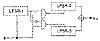Figure 16.10  Alternating stop-and-go generator.

With three LFSRs, the output generator can be written as:

b = (a1 ^ a2) ⊕ (a1 ^ a3) ⊕ (a2 ^ a3)

This is very similar to the Geffe generator, except that it has a larger linear complexity of

n1n2 + n1n3 + n2n3

where n1, n2, and n3 are the lengths of the first, second, and third LFSRs.

This generator isn’t great. Each output bit of the generator yields some information about the state of the LFSRs—0.189 bit to be exact—and the whole thing falls to a correlation attack. I don’t recommend using it.

Self-Decimated Generators

Self-decimated generators are generators that control their own clock. Two have been proposed, one by Rainer Rueppel (see Figure 16.13)  and another by Bill Chambers and Dieter Gollmann  (see Figure 16.14). In Rueppel’s generator, when the output of the LFSR is 0, the LFSR is clocked d times. When the output of the LFSR is 1, the LFSR is clocked k times. Chambers’s and Gollmann’s generator is more complicated, but the idea is the same. Unfortunately, both generators are insecure , although some modifications have been proposed that may correct the problems .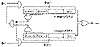Figure 16.11  Bilateral stop-and-go generator.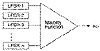Figure 16.12  Threshold generator.

Multispeed Inner-Product Generator

This generator, by Massey and Rueppel , uses two LFSRs clocked at two different speeds (see Figure 16.15). LFSR-2 is clocked d times as fast as LFSR-1. The individual bits of the two LFSRs are ANDed together and then XORed with each other to produce the final output bit of the generator.

Although this generator has high linear complexity and it possesses excellent statistical properties, it still falls to a linear consistency attack . If n1 is the length of LFSR-1, n2 is the length of the LFSR-2, and d is the speed multiple between the two, then the internal state of the generator can be recovered from an output sequence of length

n1 + n2 + log2d

Summation Generator

More work by Rainer Rueppel, this generator adds the output of two LFSRs (with carry) [1358,1357]. This operation is highly nonlinear. Through the late 1980s, this generator was the security front-runner, but it fell to a correlation attack [1053,1054,1091]. And it has been shown that this is an example of a feedback with carry shift register (see Section 17.4), and can be broken .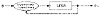Figure 16.13  Rueppel’s self-decimated generator.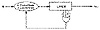Figure 16.14  Chambers’s and Gollmann’s self-decimated generator.

DNRSG

That stands for “dynamic random-sequence generator” . The idea is to have two different filter generators—threshold, summation, or whatever—fed by a single set of LFSRs and controlled by another LFSR.

First clock all the LFSRs. If the output of LFSR-0 is 1, then compute the output of the first filter generator. If the output of LFSR-0 is 0, then compute the output of the second filter generator. The final output is the first output XOR the second.

[an error occurred while processing this directive]